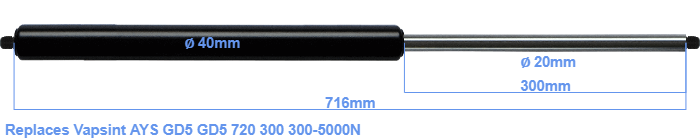# Replacement for Vapsint AYS GD5 GD5 720 300 300-5000N

£111.10 (excl. VAT)

Replacement gas spring for the Vapsint AYS GD5 GD5 720 300 300-5000 Newton. Thread: M14. Brand: Hahn.

Please note:
This replacement gas spring is 4mm shorter than the original gas spring. In general, this will not cause any problems.
 Force Choose an option300 Newton400 Newton500 Newton600 Newton700 Newton800 Newton900 Newton1000 Newton1100 Newton1200 Newton1300 Newton1400 Newton1500 Newton1600 Newton1700 Newton1800 Newton1900 Newton2000 Newton2100 Newton2200 Newton2300 Newton2400 Newton2500 Newton2600 Newton2700 Newton2800 Newton2900 Newton3000 Newton3100 Newton3200 Newton3300 Newton3400 Newton3500 Newton3600 Newton3700 Newton3800 Newton3900 Newton4000 Newton4100 Newton4200 Newton4300 Newton4400 Newton4500 Newton4600 Newton4700 Newton4800 Newton4900 Newton5000 NewtonClear